# Electronics and Communication Engineering - Exam Questions Papers

31.
Consider a stable and causal system with impulse response h[n] and rational system function H(z). Suppose it is known that H(z) contains a pole at z = 1/2 and a zero some where on the unit circle. The precise number and locations of all of the other poles is unknown.
The following statement which is false is __________ .
H(e) = 0 for some ω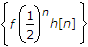g[n] = {n[h[n * h[n]} is the impulse response of a stable system
h[n] has a finite duration
Explanation:

Statement (d) is false because a finite-duration sequence must have an ROC that includes the entire z-plane, except possible z = 0 and/or z = ∞

This is not consistent with having a pole at z = 1/2.

Statement (b) is true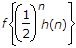corresponds to the value of the z-transform of h[n] at z = 2.

Thus, its convergence is equivalent to the point z = 2 being in the ROC.

Since the system is stable and causal, all of the poles of H(z) are inside the unit circle, and the ROC includes all the points outside the unit circle, including z = 2.

Statement (c) is true. Since the system is causal, h[n] = 0 for n < 0.

Consequently, h[n] * h[n] = 0 for n < 0; i.e., system with h[n] * h[n] as its impulse response is causal.

The same is then true for g[n] = n[h[n]].

Furthermore, by the convolution property the system function corresponding to the impulse response h[n] * h[n] is H2(z), and by the differentiation property the system function corresponding to g[n] is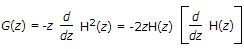From above equation we can conclude that the poles of G(z) are at the same locations as those of H(z), with the possible exception of the origin.

Therefore, since H(z) has all its poles inside the unit circle, so must G(z).

It follows that g[n] is the impulse response of a causal and stable system.

Statement (a) is true because there is a zero on the unit circle.

32.
When transient and steady state response require improvement; a __________ is used.
Lag compensator
Integrator
Explanation:

When improvement in transient state response is required Lead compensator is used.

When improvement in steady state response is required lag compensator is used.

Use of lag-lead compensator increases the low frequency gain which improves the steady state while at the same time it increases bandwidth of the system making the system response very fast.

33.
For the collector-feedback amplifier shown below the voltage gain Av will be __________ .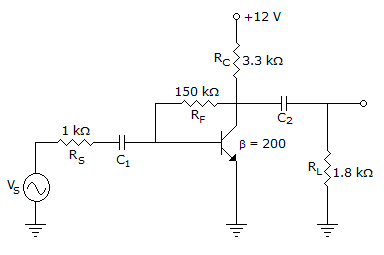-113.07
-103.07
-107.07
-93
Explanation: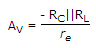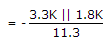= - 103.07

RF -> feedback resistor.

34.
Narrow band FM signal can be represented as
A cos (2pfct) - βA sin (2pfct) sin (2pfmt)
A cos (2pfmt) - βA sin (2pfct) sin (2pfmt)
A cos (2pfct) + βA sin (2pfct) sin (2pfmt)
A cos (2pfmt) + βA sin (2pfmt) sin (2pfct)
Explanation:

General expression for narrowband FM is

A cos (2pfct) - βA sin (2pfct) sin (2pfmt).

35.
2's complement representation of a 16 bit number (one sign bit and 15 magnitude bits) is FFFF. Its magnitude in decimal representation is
0
1
32, 767
65, 535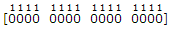→ 2s complement